# Logarithmic Spiral Tilings

Steven Dutch, Professor Emeritus, Natural and Applied Sciences, University of Wisconsin - Green Bay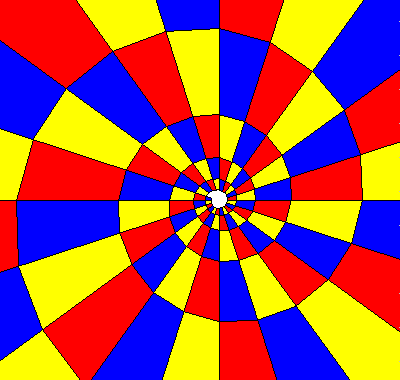A logarithmic spiral has the polar equation r=exp(ka) where r is the radius and a is the azimuth. It has the property that the curve makes a constant angle with the radius. Thus, a logarithmic spiral divided into equal radial sectors is a tesselation of geometrically similar tiles, differing only in size k is a parameter that determines how fast the spiral uncoils. If the radius increases by a factor of q per revolution, then r(a+2pi) = qr(a), exp(ka+2kpi) = q exp(ka), and, taking natural logarithms of both terms, ka + 2kpi = ln q + ka. Thus k = (ln q)/2pi.Any plane tesselation has a logarithmic spiral counterpart. The radial cells formed by the spiral and its radii correspond to the unit cells or period parallelograms of the plane tesselation. Basically a point (x,y) in the plane is mapped to point (r,a) in logarithmic spiral space. However, note that this is not a one-to-one mapping. If the radius increases by q per revolution, then point (kx,y) is mapped to (qr,a). But so is point (x,y+2pi). There can be more than one spiral: this tiling has two spirals rotated 180 degrees with respect to each other.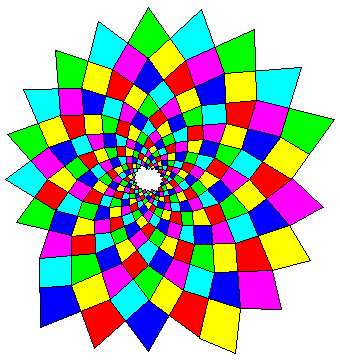Since logarithmic spiral tesselations consist of repeated motifs that grow in size, they are common growth forms in biology (sunflower heads, sea shells, etc.). This tesselation was constructed by drawing the diagonals of the square cells in the pattern above. Note that there are both clockwise and counterclockwise spirals.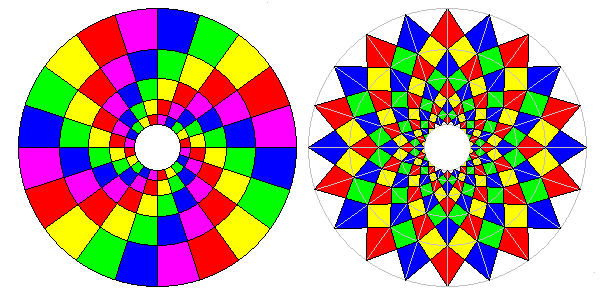It's also possible to create radial tilings consisting of circles with exponentially increasing radius. Circles are just degenerate logarithmic spirals with k=0. As the coloring shows in the left example, these tilings are still logarithmic spiral tilings. The tiling on the right was created by constructing the cell diagonals of the left example. The grid from the left example is superimposed in gray. These examples show how circular tilings are related to logarithmic spirals. If we construct a tiling with n rapidly-opening spirals and similarly placed nodes, every node will belong to a family of n nodes at the same distance from the center. We can join nodes along each spiral to create a pattern as at right, but we can also join each node to its neighbors to create a circular tiling, as shown in the left example.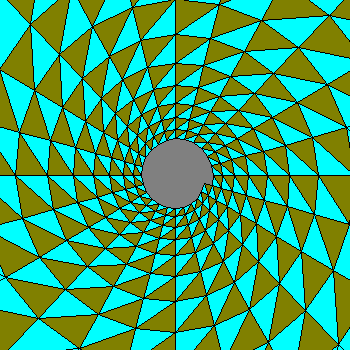If you start with a pattern of points arranged in a logarithmic spiral, and connect them to form polygons, it is a virtual certainty that the tiling will not close properly unless you calculate the spacing of points and the shape of the polygon very carefully. On the other hand, if you regard the cells formed by the spiral and its radii as period parallelograms (or unit cells) and construct the tiling with reference to the unit cells, it will always close consistently.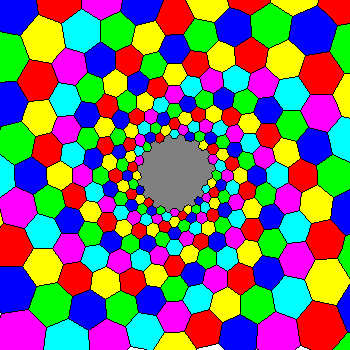A hexagon log spiral tiling. Pairs of colors outline the clockwise spirals, triplets of pastel or dark colors the counterclockwise spirals.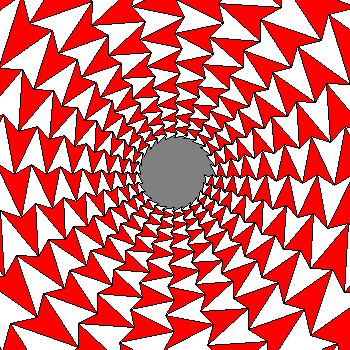Just as any quadrilateral tiles the plane, dart quadrilaterals tile the logarithmic spiral space.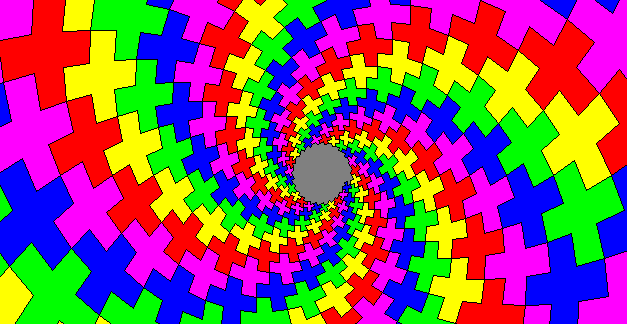This is the logarithmic spiral equivalent of the Greek Cross tiling. This is virtually impossible to create by just joining squarish radial cells of a simple log spiral tiling, but comes out perfectly if we use the square cells as period parallograms There are two ways to draw this tiling: the hard way and the easy way. The hard way needs no elaboration. The easy way is to write a computer program to plot the pattern. Page to Create Your Own Log Spiral Tesselations

Created July 1, 1999, Last Update July 21, 1999